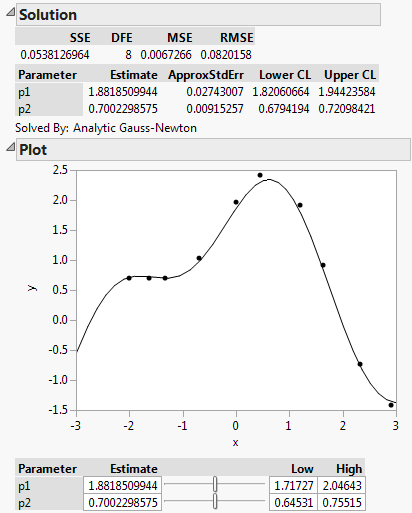Our World Statistics Day conversations have been a great reminder of how much statistics can inform our lives. Do you have an example of how statistics has made a difference in your life? Share your story with the Community!
Choose Language Hide Translation Bar
Simple nonlinear least squares curve fitting in JMP

In his Walking Randomly blog, Mike Croucher shows how to fit a simple nonlinear curve using five different statistical programming libraries: R, MATLAB, Maple, Julia and Python/numpy. The idea is to provide concrete examples for a commonly asked modeling question that is simple to state but not so simple to solve.

For a the given data set of 10 x and y values, the problem is to find the best values for parameters p1 and p2 so that the following curve best fits the y values:

p1 cos( p2 x ) + p2 sin( p1 x )

Since nonlinear fitting involves an iterative search of the solution space, we need starting parameter values, which Croucher gives as

p1=1.0, p2 = 0.2.

So how would you do the same thing in JMP?

Normally, you would start with a data table and use the Nonlinear platform under the Analyze menu to interactively launch the fit. For a model like this, which isn't built-in to JMP, you could add it in a formula column and use that in the Nonlinear dialog. But like most everything in JMP, it can be completely driven by a JSL script if you want.

Here's my JSL version:

xv= [-2, -1.64, -1.33, -0.7, 0, 0.45, 1.2, 1.64, 2.32, 2.9];

yv = [0.699369, 0.700462, 0.695354,  1.03905,  1.97389,

2.411430, 1.910910, 0.919576, -0.730975, -1.42001];

dt New Table("Data",

New Column("x", Set Valuesxv )),

New Column("y", Set Valuesyv ))

);

dt << Nonlinear( Y( :y ), X( :x ),

Model( Parameter( {p1 1, p2 0.2},

p1 *Cosine( p2 *:x ) + p2 *Sine( p1 *:x ) )),

Finish, Confidence Limits );

That script produces the following interactive output.Some of the other languages require a bit of extra work to get the confidence intervals, so it's nice to see it's just one option away in JMP.

Article Labels

There are no labels assigned to this post.